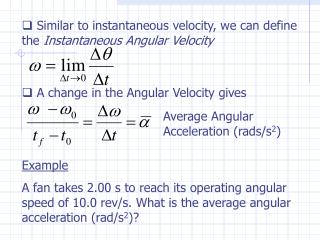Download PresentationAverage Angular Acceleration (rads/s 2 )

# Average Angular Acceleration (rads/s 2 )

Download Presentation## Average Angular Acceleration (rads/s 2 )

- - - - - - - - - - - - - - - - - - - - - - - - - - - E N D - - - - - - - - - - - - - - - - - - - - - - - - - - -
##### Presentation Transcript

1. Similar to instantaneous velocity, we can define the Instantaneous Angular Velocity • A change in the Angular Velocity gives Average Angular Acceleration (rads/s2) Example A fan takes 2.00 s to reach its operating angular speed of 10.0 rev/s. What is the average angular acceleration (rad/s2)?

2. Solution: Given: tf=2.00 s, f=10.0 rev/s Recognize: t0=0, 0=0, and that f needs to be converted to rads/s

3. Equations of Rotational Kinematics • Just as we have derived a set of equations to describe ``linear’’ or ``translational’’ kinematics, we can also obtain an analogous set of equations for rotational motion • Consider correlation of variables • TranslationalRotational • x displacement  • v velocity  • a acceleration  • t time t

4. Replacing each of the translational variables in the translational kinematic equations by the rotational variables, gives the set of rotational kinematic equations • We can use these equations in the same fashion we applied the translational kinematic equations

5. Example: Problem 8.16 A figure skater is spinning with an angular velocity of +15 rad/s. She then comes to a stop over a brief period of time. During this time, her angular displacement is +5.1 rad. Determine (a) her average angular acceleration and (b) the time during which she comes to rest. Solution: Given: f=+5.1 rad, 0=+15 rad/s Infer: 0=0, f=0, t0=0 Find: , tf ?

6. (a) Use last kinematic equation (b) Use first kinematic equation

7. Or use the third kinematic equation Example: Problem 8.26 At the local swimming hole, a favorite trick is to run horizontally off a cliff that is 8.3 m above the water, tuck into a ``ball,’’ and rotate on the way down to the water. The average angular speed of rotation is 1.6 rev/s. Ignoring air resistance,

8. determine the number of revolutions while on the way down. y v0 y0  yf Solution: Given: 0 = f = 1.6 rev/s, y0 = 8.3 m Also, v0y = 0, t0 = 0, yf = 0 Recognize: two kinds of motion; 2D projectile motion and rotational motion with constant angular velocity. Method: #revolutions =  = t. Therefore, need to find the time of the projectile motion, tf. x

9. Consider y-component of projectile motion since we have no information about the x-component.

10. Tangential Velocity and Acceleration • For one complete revolution, the angular displacement is 2 rad • From our studies of Uniform Circular Motion (Chap. 5), we know that the time for a complete revolution is a period T  v r  z • Therefore the angular frequency can be written

11. Also from Chap. 5, we know that the speed for an object in a circular path is Tangential velocity (rad/s) • The tangential velocity corresponds to the speed of a point on a rigid body, a distance r from its center, rotating at an angular velocity  vT • Each point on the rigid body rotates at the same angular velocity, but its tangential speed depends on its location r r r=0